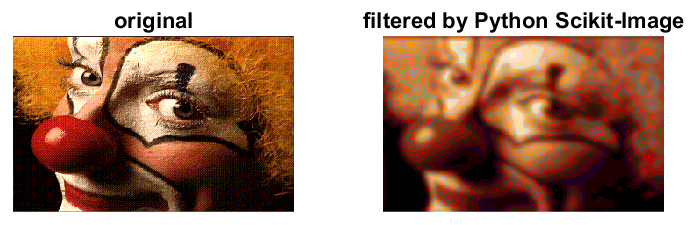## Matlab matrices &harr; Python

Related:

Passing N-dimensional arrays to/from Python from Matlab is straightforward with Matlab ≥ R2018b. Matlab understands lists, dicts, sets, scalars, and other less frequently used classes from Python. Matlab R2018b supports Python versions 2.7, 3.5 and 3.6.

## Matlab → Python

Using image_proc.m:1. Load an image in Matlab 2. filter the image in Python 3. display result in Matlab.

## Matlab ↔ xarray

These functions are used to access the auroral model GLOW from Matlab via glow.m.

``````function M = xarray2mat(V)
M = double(py.numpy.asfortranarray(V));
end

function I = xarrayind2vector(V,key)
% convert xarray index to Matlab 1-D matrix

I = cell2mat(cell(V.indexes{key}.values.tolist));

end
``````